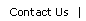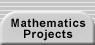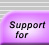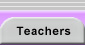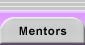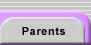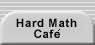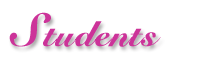From 1999-2002, Making Mathematics connected students with professional mathematicians to work on fun, interesting, and challenging math research projects. Working alongside a Making Mathematics mentor, students experienced and learned the methods that mathematicians use in their work, saw mathematics as a scientific discipline, and saw what mathematicians really do every day.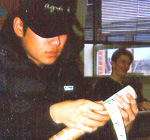Mathematics research projects are longer-term problems that are more open-ended and require deeper investigation than the shorter problems most students encounter in mathematics classes. Students had the power to take a project in their own directions (ones that interested them, rather than ones that were assigned to them).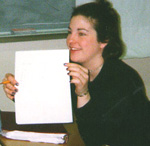Making Mathematics mentors were mathematicians who gave guidance and assistance over email. Students and mentor emailed each other (about once a week) concerning a particular mathematics problem. Students described the work they did on the project, answered and asked questions, shared solutions, and exchanged resources for finding new solutions. When their work was complete, they learned how to show their work and thought processes to others, and they also saw how others might learn from their work.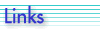by Joe Noss joenoss@ioe.ac.uk 6.1 student at a school near London, England I used to think of maths, (for reasons I don’t understand in the US you only have one?) as being a bit like cookery. It consisted of "recipes": self contained nucleuses of technique, which gave me the tools to answer particular types of problems.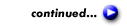Dan McGinn (PDF)  Eric Landquist (PDF)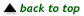Translations of mathematical formulas for web display were created by tex4ht. © Copyright 2003 Education Development Center, Inc. (EDC)